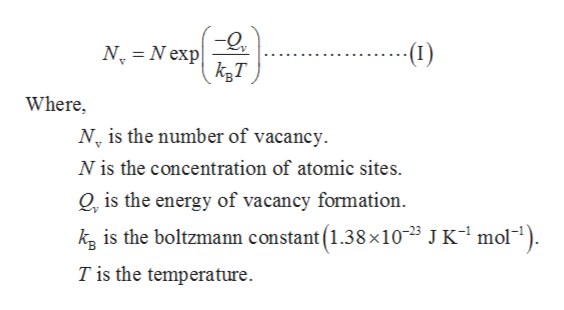# The number of vacancies in some hypothetical metal increases by a factor of 5 when the temperature is increased from 1060 K to 1160 K. Calculate the energy (in kJ/mol) for vacancy formation assuming that the density of the metal remains the same over this temperature range.

Question
439 views

The number of vacancies in some hypothetical metal increases by a factor of 5 when the temperature is increased from 1060 K to 1160 K. Calculate the energy (in kJ/mol) for vacancy formation assuming that the density of the metal remains the same over this temperature range.

check_circle

Step 1

The presence of vacancy in a crystal is a kind of point defect. The number of vacancies in a crystal is calculated by the formula shown below.

Step 2

Given data:

Step 3

The energy for the vacancy formation is cal...help_outlineImage Transcriptionclose-(1) N N exp 11 Where, N is the number of vacancy N is the concentration of atomic sites Q, is the energy of vacancy formation. kg is the boltzmann constant (1.38x105 J K1 mol-1). T is the temperature fullscreen

### Want to see the full answer?

See Solution

#### Want to see this answer and more?

Solutions are written by subject experts who are available 24/7. Questions are typically answered within 1 hour.*

See Solution
*Response times may vary by subject and question.
Tagged in